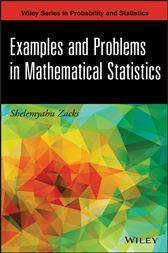# Examples and Problems in Mathematical Statistics

## Series: Wiley Series in Probability and StatisticsCA\$173.50
CA\$156.15
###### ISBNs
• 1118606000
• 9781118605509
• 9781118606001
• 9781118605837

Provides the necessary skills to solve problems in mathematical statistics through theory, concrete examples, and exercises

With a clear and detailed approach to the fundamentals of statistical theory, Examples and Problems in Mathematical Statistics uniquely bridges the gap between theory andapplication and presents numerous problem-solving examples that illustrate the relatednotations and proven results.

Written by an established authority in probability and mathematical statistics, each chapter begins with a theoretical presentation to introduce both the topic and the important results in an effort to aid in overall comprehension. Examples are then provided, followed by problems, and finally, solutions to some of the earlier problems. In addition, Examples and Problems in Mathematical Statistics features:

• Over 160 practical and interesting real-world examples from a variety of fields including engineering, mathematics, and statistics to help readers become proficient in theoretical problem solving
• More than 430 unique exercises with select solutions
• Key statistical inference topics, such as probability theory, statistical distributions, sufficient statistics, information in samples, testing statistical hypotheses, statistical estimation, confidence and tolerance intervals, large sample theory, and Bayesian analysis

Recommended for graduate-level courses in probability and statistical inference, Examples and Problems in Mathematical Statistics is also an ideal reference for applied statisticians and researchers.

• Wiley; December 2013
• ISBN: 9781118606001
• Edition: 1
• Title: Examples and Problems in Mathematical Statistics
• Series: Wiley Series in Probability and Statistics
• Author: Shelemyahu Zacks
• Imprint: Wiley
###### ISBNs
• 1118606000
• 9781118605509
• 9781118606001
• 9781118605837